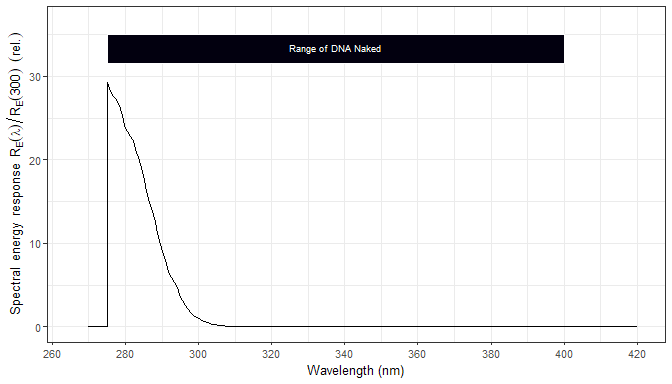# User Guide: 2 Plot Methods

## Introduction

Package ggspectra extends ggplot2 with stats, geoms and annotations suitable for light spectra. It also defines ggplot() and plot() methods specialized for the classes defined in package photobiology for storing different types of spectral data. This vignette describes the use of these plot methods.

Although the package uses internally ggplot2, photobiology and photobiologyWavebands it does not re-export these packages, which need to loaded if used.

library(ggplot2)
library(dplyr)
##
## Attaching package: 'dplyr'
## The following object is masked from 'package:ggplot2':
##
##     vars
## The following objects are masked from 'package:stats':
##
##     filter, lag
## The following objects are masked from 'package:base':
##
##     intersect, setdiff, setequal, union
library(photobiology)
library(photobiologyWavebands)
library(ggspectra)

We bind two spectra into an object to be used later to demonstrate grouping.

two_suns.spct <- rbindspct(list(sun1 = sun.spct, sun2 = sun.spct * 2))

We change the default theme.

theme_set(theme_bw(10))

## Plotting spectra

The most automatic way of plotting spectral data stored in one of the classes defined in package photobiology is to use the plot()methods. These go much further than the plot() method defined in base-R for numeric data, as these classes store metadata that allows the automatic construction of axis labels as quantities and units are well defined.

Here we use the same example source_spct object from package photobiology used in the User Guide. In contrast to the examples in the User Guide, here we obtain with a very simple statement a complete annotated plot of the solar spectrum at ground level.

plot(sun.spct)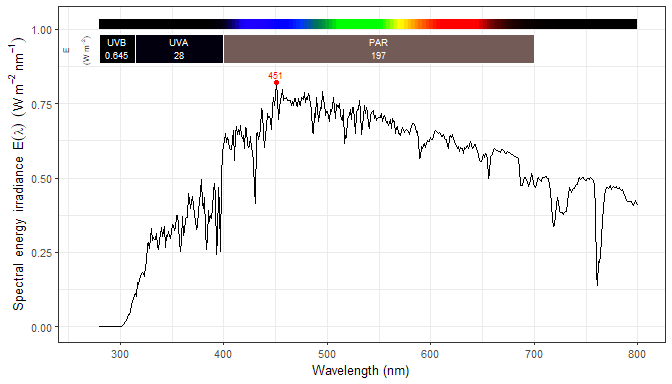Although the defaults provide a useful plot, the plot() methods accepts arguments for several parameters that allow to tweak the looks and contents of the plots without explicit manipulation of the spectral data.

### Summary quantities in labels

In this first example we pass "mean" as argument to label.qty, to print means instead of integrals in the labels. Note that the units and quantity labels for the waveband summaries have also changed.

plot(sun.spct, label.qty = "mean")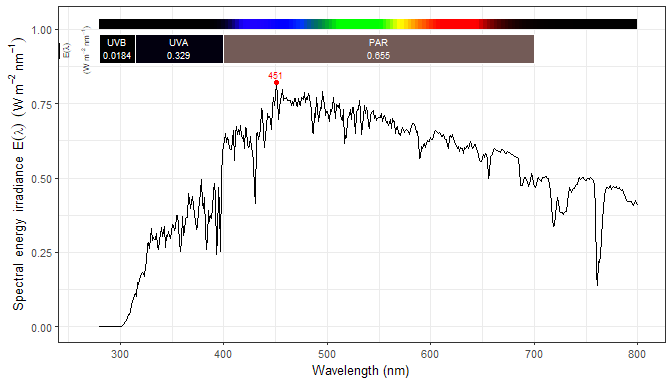Two label.qty values need explanation. The first one, "relative" displays in labels the relative contribution of the integral of each waveband, to the sum of the integrals of all wavebands. The second one, "contribution" displays in labels the integral of each waveband divided by the integral of the whole spectrum. Consequently, this second option should be interpreted with caution, as the spectral data is unlikely in many cases to include the whole emission or absorption spectrum of a source.

plot(sun.spct, label.qty = "contribution")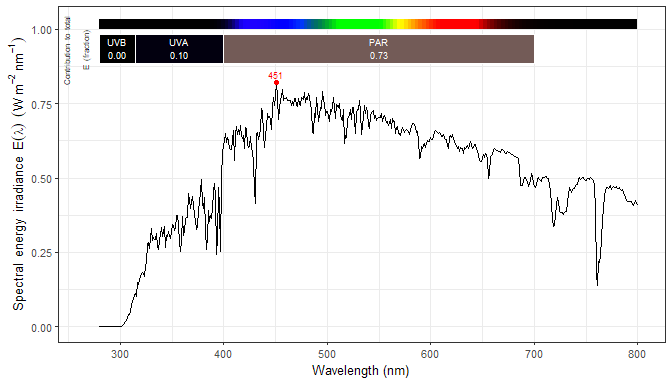plot(sun.spct, label.qty = "relative")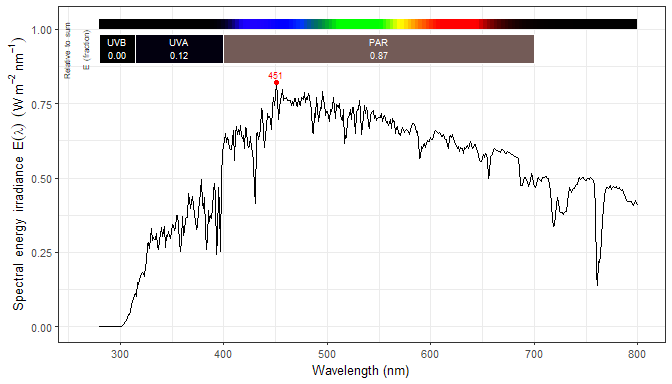plot(sun.spct, label.qty = "mean", unit.out = "photon")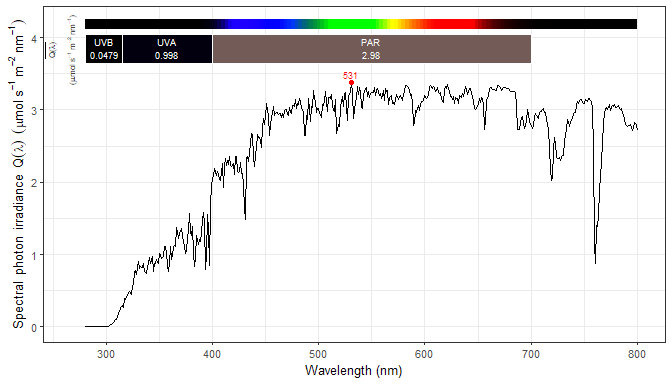plot(sun.spct, label.qty = "relative.pc")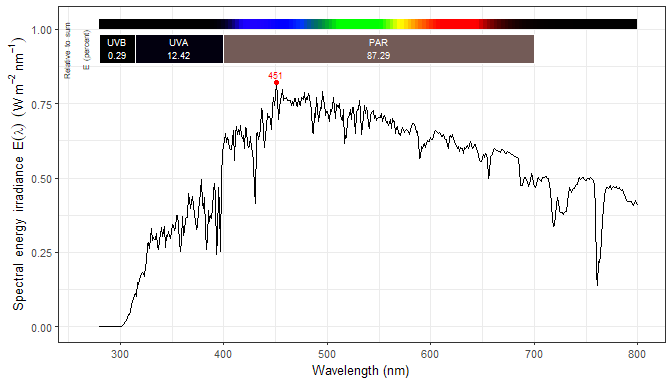We can change the basis of expression of spectral irradiance and irradiance from "energy" to "photon". Be aware that by design, all values, summaries and spectral data will always use the same base of expression.

plot(sun.spct, unit.out = "photon")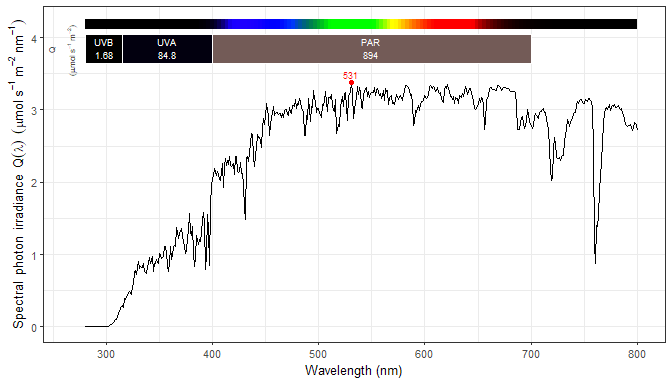### Automatic annotations

Which annotations are included can be controlled through parameter annotations. These are passed as a vector of character strings, with three values with special meaning for the first element (with index = 1): "=" means use only the annotations that follow in the vector, "+" means add to the default the annotations that follow, and "-" means remove the annotations that follow from the defaults. A NULL value means use defaults as is, "" means no annotations, and "reserve.space" means no annotations, but expand axis limits and set identity scales ready for manually adding annotations.

annotation default for classes overrides
“boxes” all
“segments” none “boxes”
“color.guide” all
“peaks” all
“peak.labels” none “peaks”
“valleys” none
“valley.labels” none “valleys”
“labels” all
“summaries” source_spct, response_spct, filter_spct, reflector_spct
“boundaries” raw_spct
“title” none
“title:type none
“title:type:type none

Titles come in different flavours. Package ‘ggplot2’ now supports both titles and subtitles. The "title" as argument to parameter annotation of plot() methods and of function ggtitle_spct() takes optional modifiers that can be used to specify the contents of the automatic title and subtitle. Currently supported modifiers are shown in the table below. If the metadata item is not stored in the plotted spectral object, the title or subtitle will show NA. See the documentation of packages ‘photobiology’ and ‘ooacquire’ for information on how to set and unset attributes of spectral objects. To add an arbitrary title and/or subtitle to a plot, use either function ggtitle() or function labs() from package ‘ggplot2’.

Modifier text source used
objt name of the object plotted
class class of the plotted object
what what.measured attribute
when when.measured attribute
where where.measured attribute
inst.name spectrometer name
inst.sn spectrometer serial number
none no title or no subtitle

For example "title:objt", the default for "title", adds a title with the name of the object being plotted. what, when and where use these three attributes if available. For example "title:what:when" will use thewhat.measuredattribute for the title and thewhen.measured attribute for the subtitle.

We can add an annotation to the defaults.

plot(sun.spct,
annotations = c("+", "title:when:where"))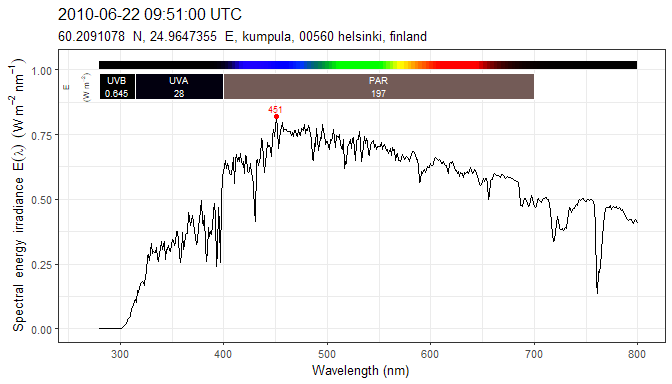In this case "boundaries", an annotation that adds a horizontal dashed line and does not override any other annotation.

plot(sun.spct,
annotations = c("+", "boundaries"))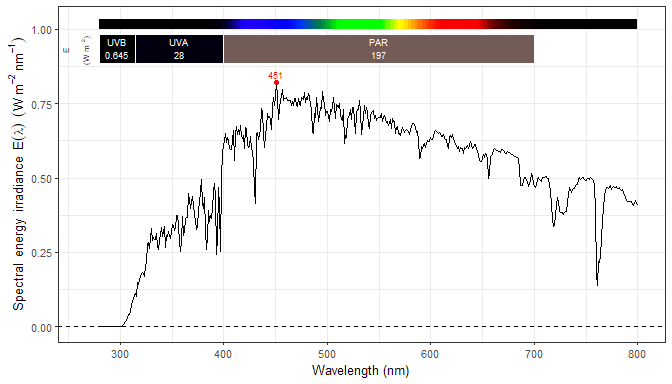We can list all the annotations to be included in a plot, in which case "=" is optional so as to maintain compatibility with earlier versions.

plot(sun.spct,
annotations = c("=", "labels", "summaries", "color.guide", "peaks", "boundaries"))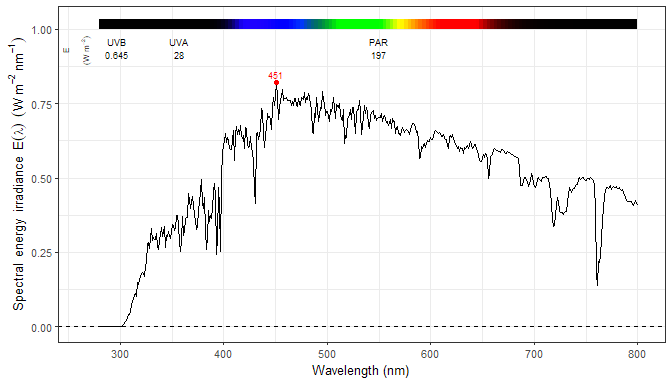As indicated in the table above, some annotations override other annotations which fulfil a similar role. Here "segments" overrides "boxes", in the default.

plot(sun.spct,
annotations = c("+", "segments"))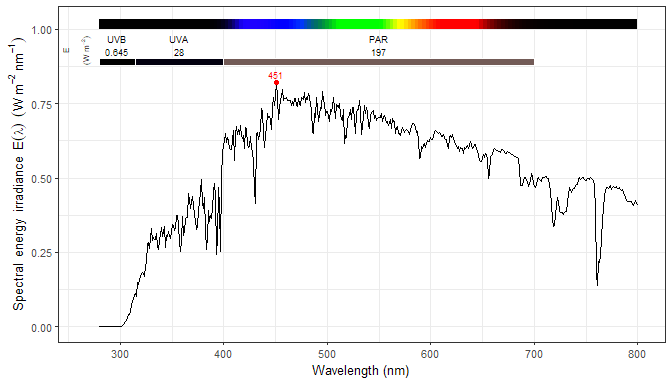We can also remove some of the default annotations.

plot(sun.spct, annotations = c("-", "summaries", "peaks"))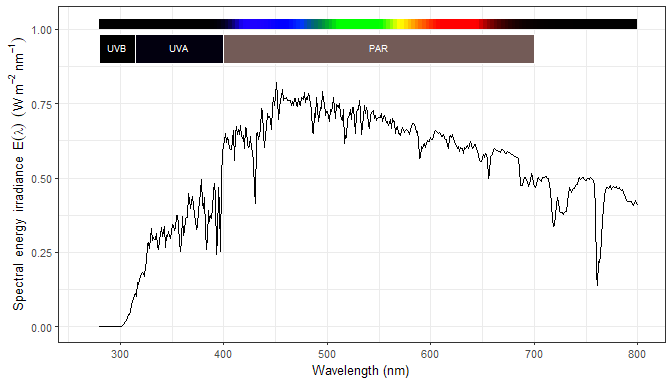We add "valleys" as annotations, and control with span how near peaks and valleys are found.

plot(sun.spct, annotations = c("+", "valleys"), span = 41)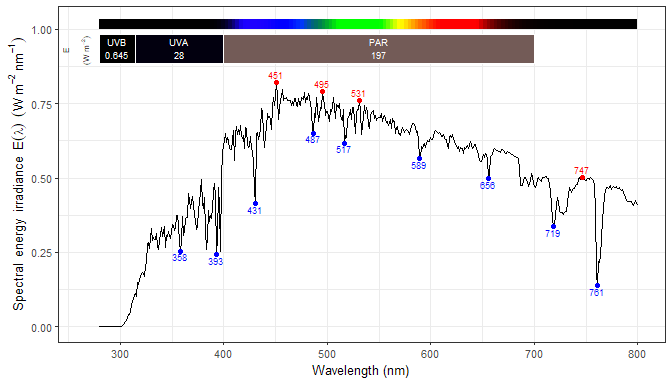The annotations "peak.labels" and "valley.labels" override "peaks" and "valleys". They use the repulsive geometry geom_label_repel from package ‘ggrepel’.

plot(sun.spct, annotations = c("+", "peak.labels", "valley.labels"), span = 71)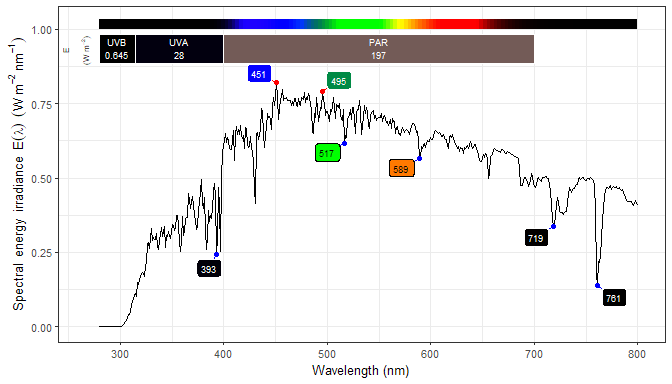Passing "" as argument to annotations results in a plot with no annotations, and no scales set.

plot(sun.spct, annotations = "")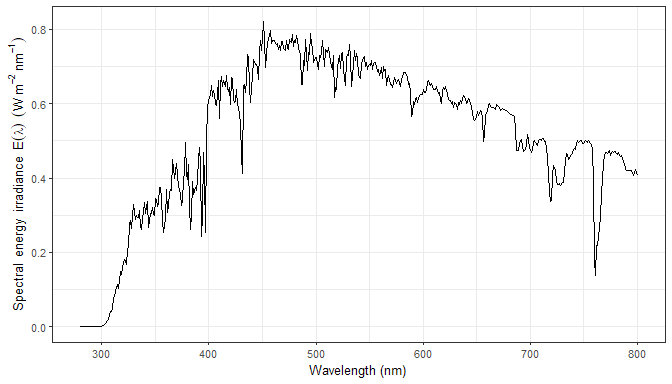Passing "reserve.space" as argument to annotations results in a plot with no annotations, but scales set and expanded so as to receive annotations.

plot(sun.spct, annotations = "reserve.space")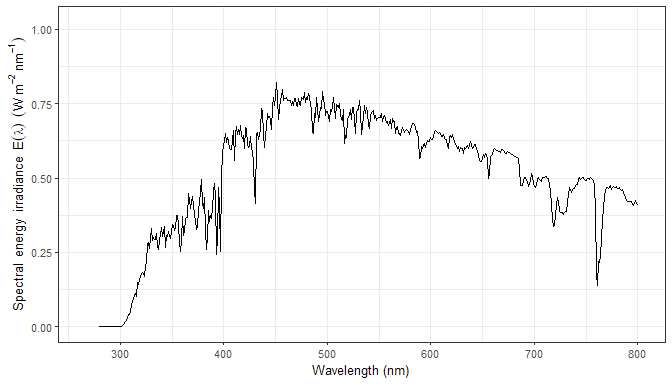The size of the font used for the annotations is controlled by argument text.size.

plot(sun.spct, annotations = c("=", "segments", "labels", "color.guide"),
text.size = 3.5)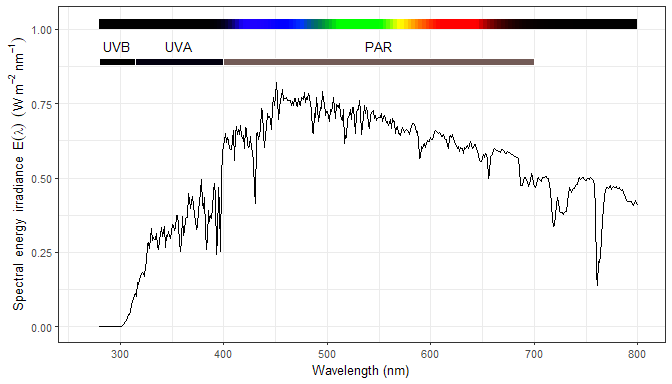Arguments range and w.band play very different roles. The first one determines the range of wavelengths to include in the data plotted, which is slightly different to the effect of ggplot2::xlim() as range is used to trim the spectral data before passing it to ggplot, using interpolation when needed (see photobiology::trim_wl()). The second one, w.band is only used for the annotations and decorations. It should be however noted, that a waveband object is a valid argument for both range and w.band.

plot(sun.spct, range = VIS())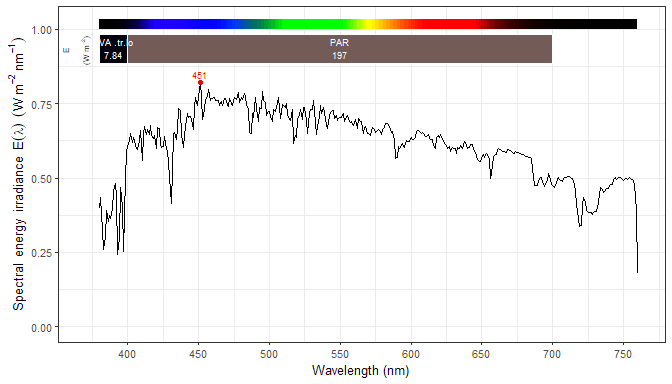plot(sun.spct, w.band = PAR())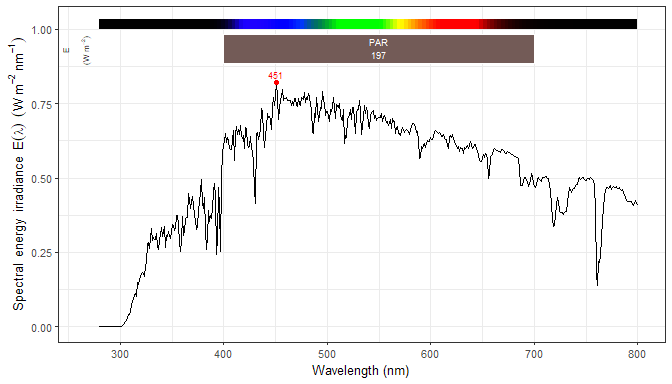plot(sun.spct, w.band = CIE())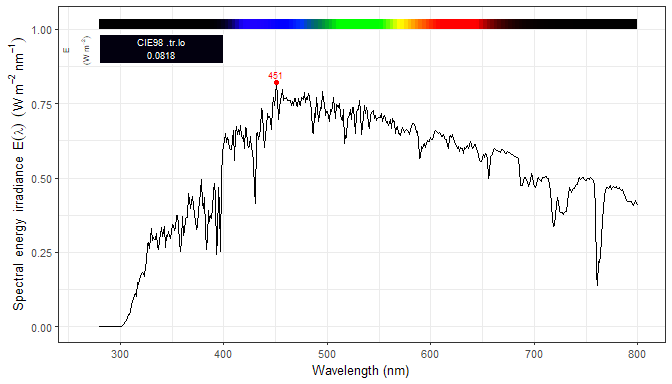NULL as argument for w.band is replaced by a waveband covering the full range of the spectral data.

plot(sun.spct, w.band = NULL)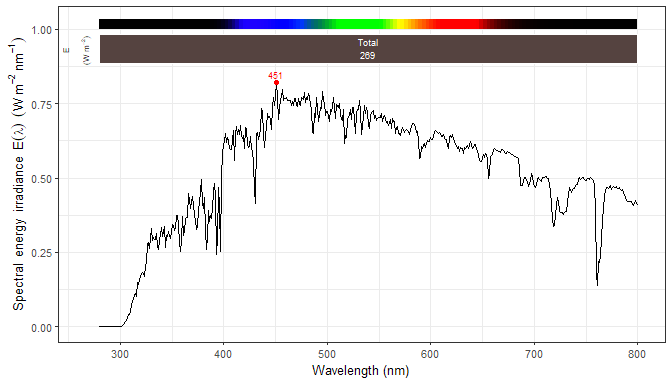The whole range is the range plotted, which is controlled by the argument passed to range.

plot(sun.spct, w.band = NULL, range = c(400,700))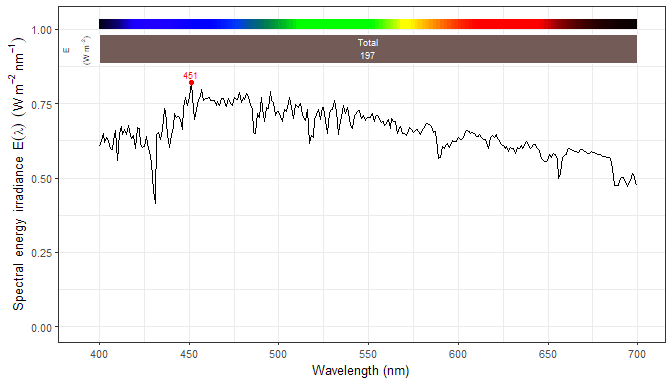The argument passed to range can be a waveband or and spectrum, in which case their wavelength range is used.

plot(sun.spct, w.band = NULL, range = PAR())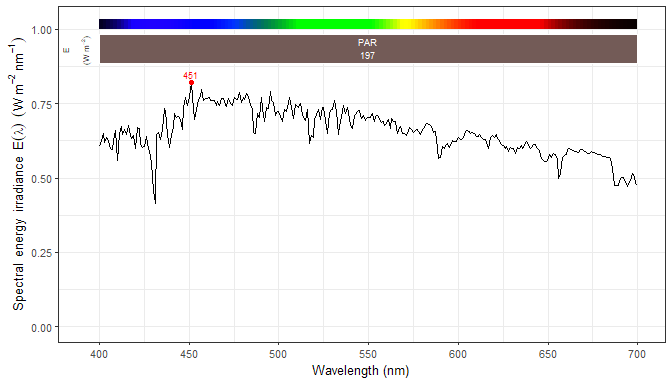plot(sun.spct, w.band = PAR(), range = PAR())plot(sun.spct, w.band = VIS_bands(), range = VIS())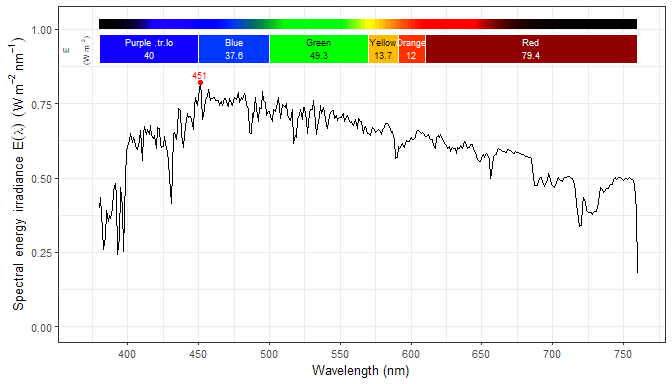The time unit is also stored in the metadata, as demonstrated here. The units in axis labels have changed to the units used in the spectral data.

getTimeUnit(sun.daily.spct)
##  "day"
plot(sun.daily.spct)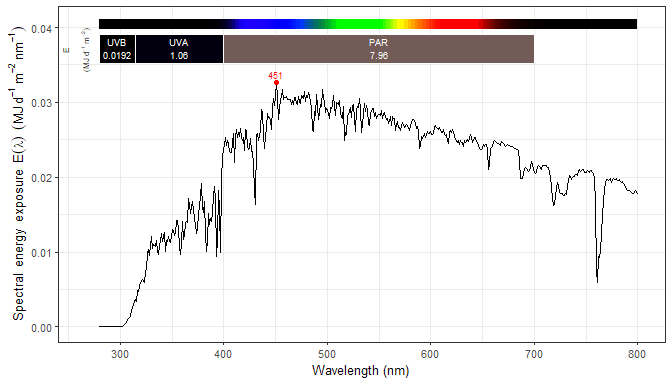Even though the plot() methods can return a finished plot, the returned object is a ggplot object and can be built upon by adding additional elements like facets, aesthetics and even additional layers.

plot(two_suns.spct, label.qty = "mean") + facet_wrap(~spct.idx)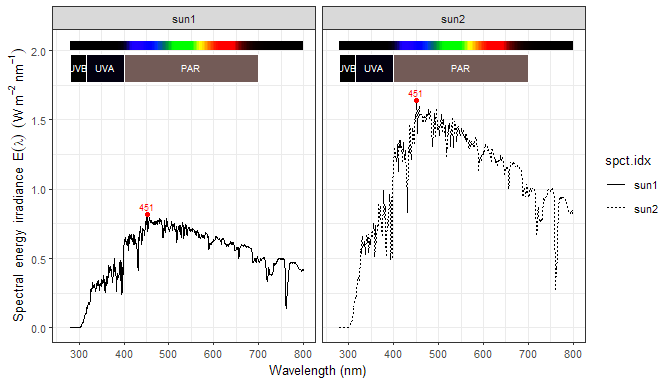When using grouping the "summaries" annotation would be over-plotted, and needs to be suppressed.

plot(two_suns.spct, annotations = c("-", "summaries")) +
aes(linetype = spct.idx)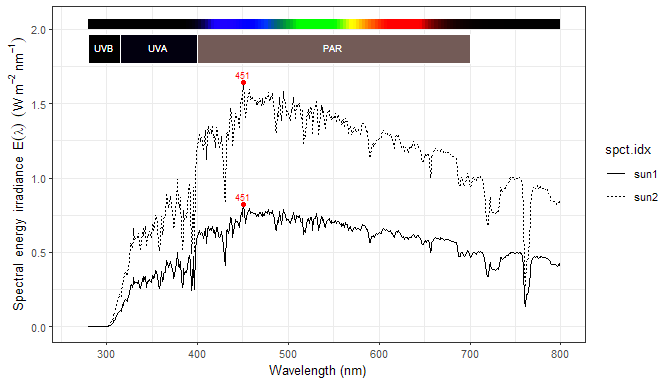It is possible to construct and bind the spectra on-the-fly.

plot(rbindspct(list(sun = sun.spct, filtered = yellow_gel.spct * sun.spct)),
annotations = c("-", "summaries")) +
aes(linetype = spct.idx)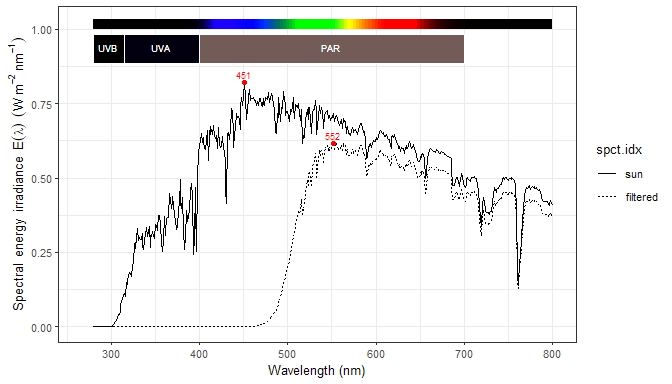In the examples above the source_spct object sun.spct was used. The plot() methods for other spectral classes have slight differences. We show some examples for filter_spct objects.

plot(yellow_gel.spct, annotations = c("-", "peaks"))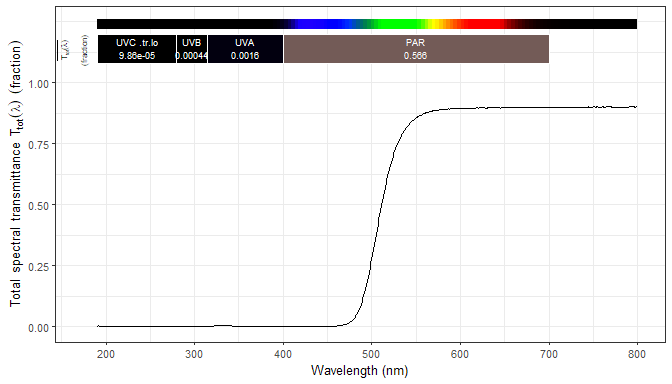By default transmittance, reflectance and absorptance are expressed as fractions of one, as in the plot above, but optionally percents can be plotted.

plot(yellow_gel.spct, pc.out = TRUE, annotations = c("-", "peaks"))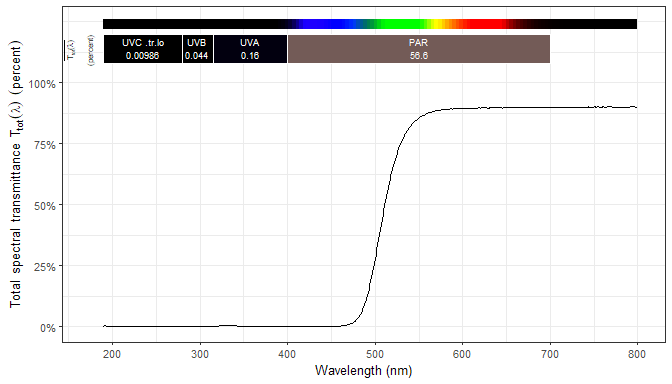In many cases it is possible to convert on-the-fly the quantity plotted.

plot(yellow_gel.spct, plot.qty = "absorbance", annotations = c("-", "peaks"))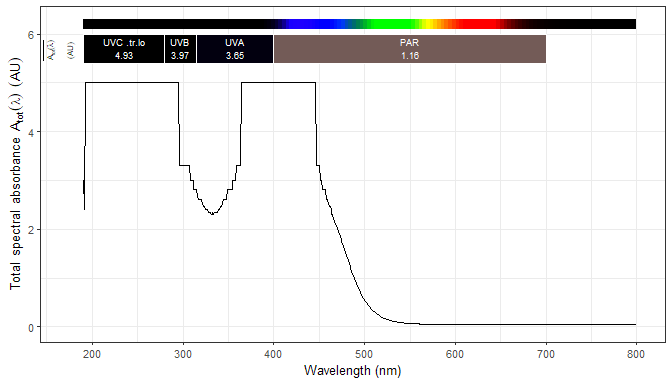We add a guessed spectral reflectance to the data object to allow the estimation of internal absorptance.

yellow_gel.spct$Rfr <- 1 - max(yellow_gel.spct$Tfr)
plot(yellow_gel.spct, plot.qty = "absorptance", annotations = c("-", "peaks"))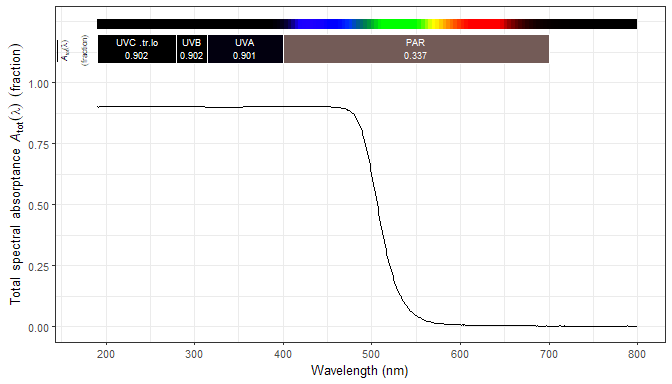If one really needs to, one can add a suitable stat using ‘local’ data. A peak annotation could be added manually.

plot(sun.spct) + geom_spct(fill = color_of(sun.spct)) +
geom_spct(data = yellow_gel.spct * sun.spct, color = "black",
fill = color_of(yellow_gel.spct * sun.spct))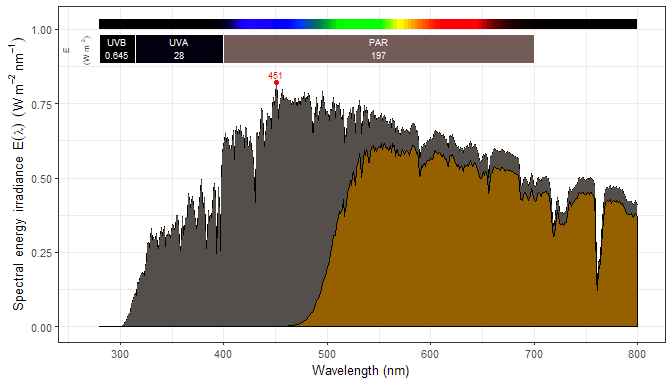The examples above use an emission spectrum. All the spectral classes defined in package ‘photobiology’ are supported, however.

plot(yellow_gel.spct)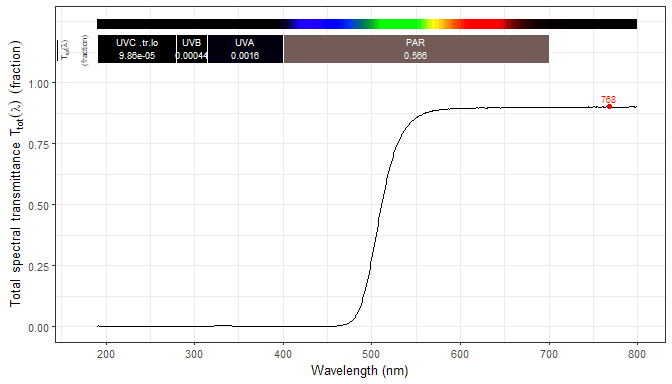In the case of quantities like transmittance which have a certain range of valid values, both upper and lower boundaries are highlighted.

plot(yellow_gel.spct, annotations = c("+", "boundaries"))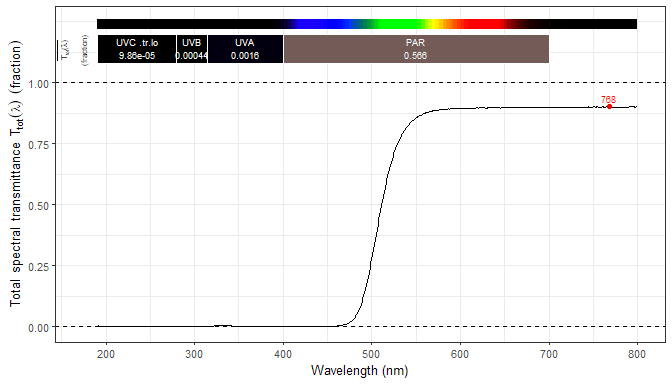In the case of raw instrument counts data, if the spectral object contains an instrument descriptor as metadata, the upper boundary is set to the maximum counts of the detector.

plot(white_led.raw_spct, annotations = c("+", "boundaries"))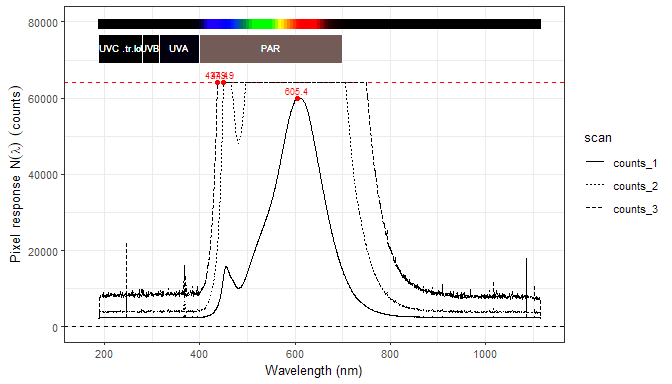Both raw_spct and cps_spct objects contain multiple data columns when integration time bracketing has been used during data acquisition. In such cases, if one wants to plot only one of the raw spectra, method select() from package ‘dplyr’ should be used instead of subscripting ([ ]) to preserve the metadata.

plot(select(white_led.raw_spct, w.length, counts_1),
annotations = c("+", "boundaries"))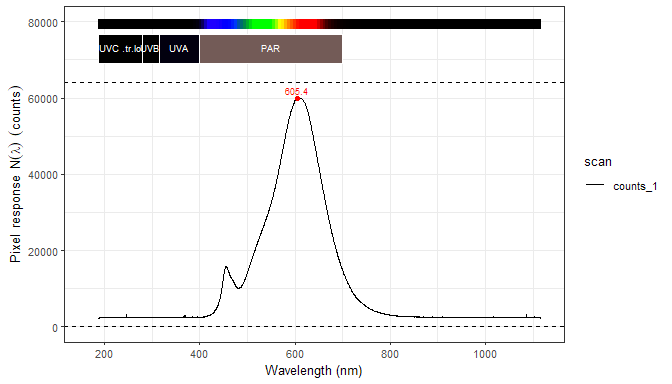### Handling of off-range data

If the supplied data include off-range values such as negative irradiance or fractional transmittance, reflectance, or absorptance outside the zero to one range the exceeded boundary is highlighted.

plot(yellow_gel.spct - 0.01)
## Warning in range_check_Tfr(x, strict.range = strict.range): Off-range
## transmittance values [-0.00999..0.89250] instead of [0..1]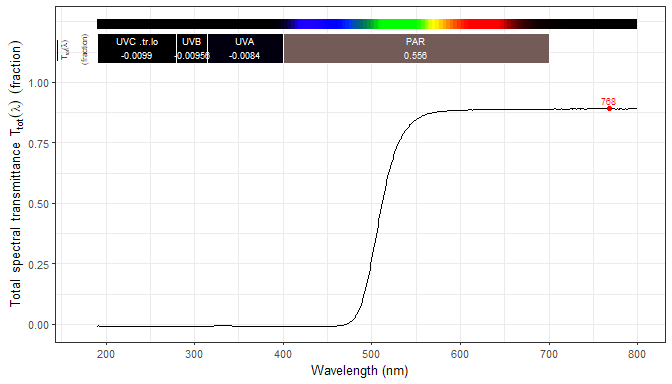## Ploting collections of spectra

A plot() is available for collections of spectra. We use in the examples a simple collection of two spectra.

two_suns.mspct <- source_mspct(list(sun1 = sun.spct, sun2 = sun.spct / 2))
mixed.mspct <- generic_mspct(list(sun = sun.spct, filter = polyester.spct))

Plot accepting all defaults.

plot(two_suns.mspct)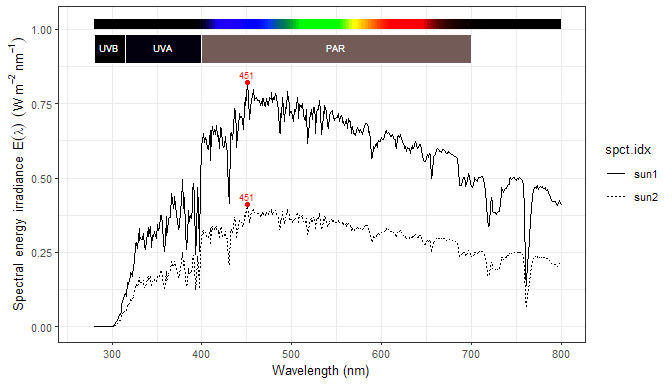Plot using one panel per spectrum.

plot(two_suns.mspct, annotations = c("+", "summaries")) +
facet_wrap(~spct.idx, ncol = 1)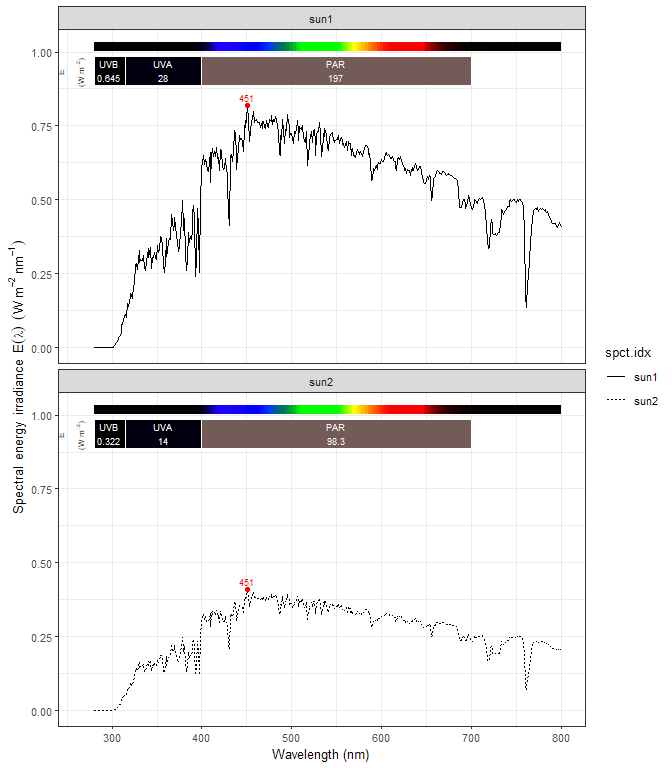Plot as separate plots. This approach is specially useful with heterogeneous generic_mspct objects, although it works with any collection of spectra.

multiplot(plotlist = mslply(mixed.mspct, plot))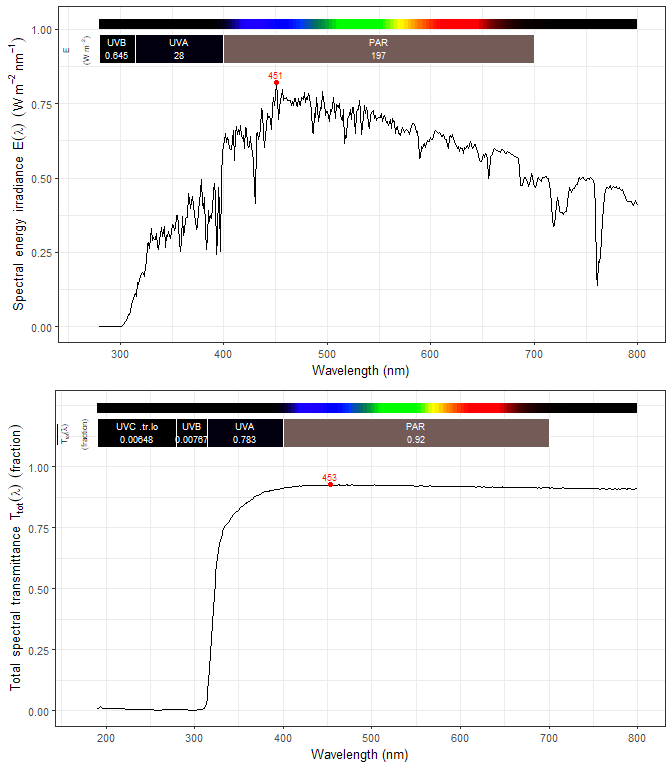Using colors is slightly more involved as the identity scale is in use for color in annotations. For this same reason no color key in generated automatically. (In the case of more than two spectra it is easier to use plyr::mapvalues instead of several nested ifelse calls.)

plot(two_suns.mspct) +
aes(color = ifelse(spct.idx == "sun1", "darkgreen", "darkred"))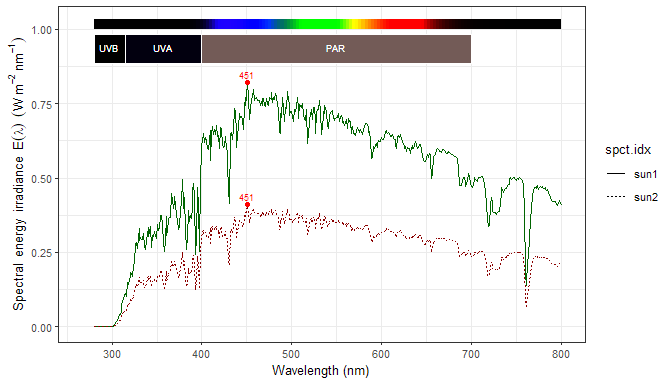Plots created with plot() method are ggplot objects and can be customized, bearing in mind that any added layers will be plotted on top or existing layers.

plot(two_suns.mspct, annotations = c("color.guide", "valleys", "peaks"), span = 51)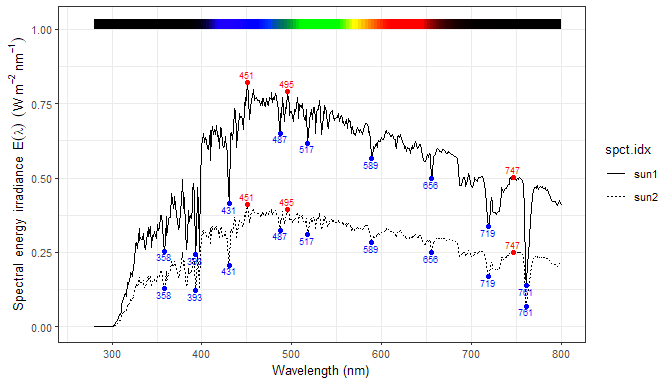Here we replace the default peaks annotations with a custom one, but still take advantage of other defaults like nice axis labels and other annotations.

plot(two_suns.mspct, annotations = c("-", "peaks")) +
stat_peaks(span = NULL, color = "red") +
stat_peaks(span = NULL, geom = "text",
label.fmt = "max at %3.1f nm",
vjust = -0.4, color = "red")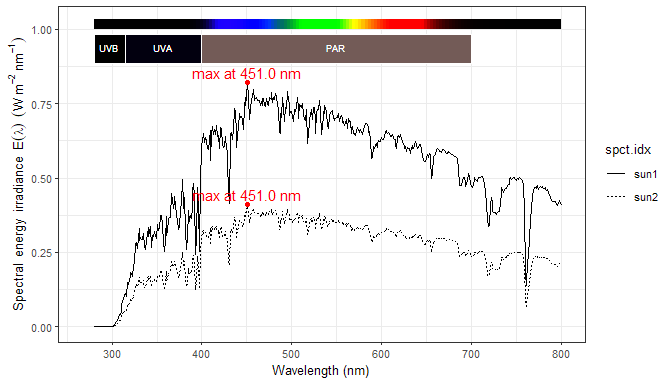## Ploting wavebands

A plot() method for waveband objects is also provided.

plot(VIS())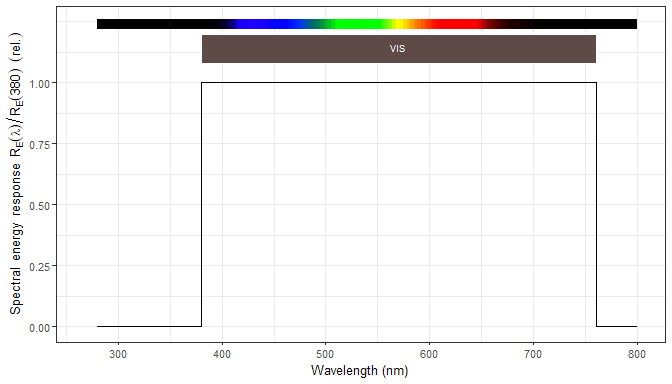plot(CIE(), range = CIE(), annotations = c("-", "color.guide"))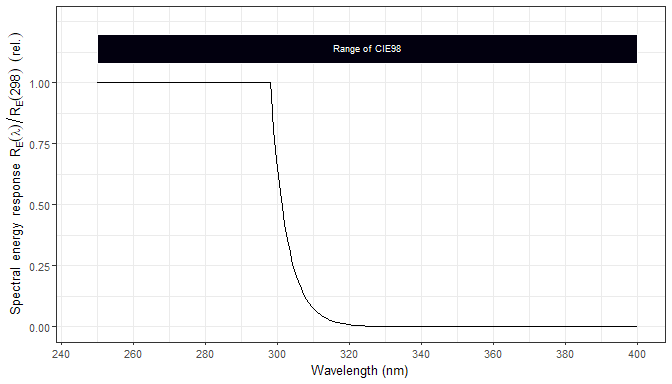plot(DNA_N(), range = c(270, 420), annotations = c("-", "color.guide"))`